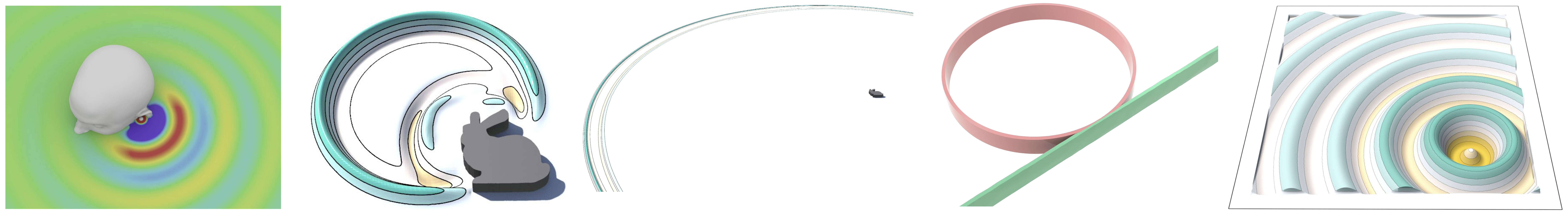### Research

##### Overview
My research covers mathematical modeling and numerical simulation of acoustic systems. My approach to modeling and simulation is guided by several core concepts in differential geometry, including conformal invariance, differential forms, and geometric variational principles. These geometric tools can be used to overcome many difficulties and limitations that exist in standard Newtonian models and coordinate-based Euclidean spaces. Currently, I am investigating applications of this geometric approach in three areas of acoustic simulation: geometric boundary modeling, non-spherical harmonics, and cochlear fluid dynamics. My research has potential applications in a variety of fields including virtual acoustics, music technology, and hearing science.

##### Geometric Boundary Modeling for Acoustic Wave Simulation
I have developed differential geometric methods for handling boundaries in acoustic wave simulations, including the Minkowski-Kelvin transformation and discrete perfectly matched layers. A promising future direction of my research involves developing boundary flattening methods for curved obstacles. A common theme in this work is the discovery of conformally invariant transformations of wave propagation, an approach that derives from Felix Klein's Erlangen program. Application areas include spatial audio, architectural acoustics, artificial reverberation, and hearing devices.##### Non-Spherical Harmonics for Acoustic Simulation, Capture, and Rendering
Below is an image of four of the harmonic modes of the Stanford bunny mesh. From left to right, I've plotted the $$0^{th}$$, $$1^{st}$$, $$2^{nd}$$, and $$6^{th}$$ modes. In this methodology, I compute the displacement of each mode by numerically solving an eigen value problem at every vertex on the triangulated mesh. The purple portions represent regions where the displacement is maximally negative, and the red portions represent where it is maximally positive. Notably, the solution is computed using coordinate-free differential forms, which allow one to more easily model dynamics on arbitrary geometries. I am interested in using this methodology to calculate acoustic transfer functions involving complex rigid obstacles geometries, such as head-related transfer functions, and to capture and render sounds using non-spherical microphone arrays.##### Physical Simulation of Cochlear Fluid Dynamics
Physical simulation is the computational emulation of natural phenomena using physics-based models. Physical simulation is useful for producing very accurate emulations and offering intuitive user control of modeling parameters. In the past, I have developed physics-based modal synthesis and finite-difference time-domain simulations of musical instruments and human speech. Currently, I am interested in using geometric variational principles to simulate fluid-solid interactions in the human cochlea.